• 在没有猫的训练集上训练出来一个图片分类器，这个分类器需要在看过少数几张猫的照片后分辨出测试集的照片中有没有猫。
• 训练一个玩游戏的AI，这个AI需要快速学会如何玩一个从来没玩过的游戏。
• 一个仅在平地上训练过的机器人，需要在山坡上完成给定的任务。

## 元学习问题定义

### A Simple View

$\theta^* = \arg\min_\theta \mathbb{E}_{\mathcal{D}\sim p(\mathcal{D})} [\mathcal{L}_\theta(\mathcal{D})]$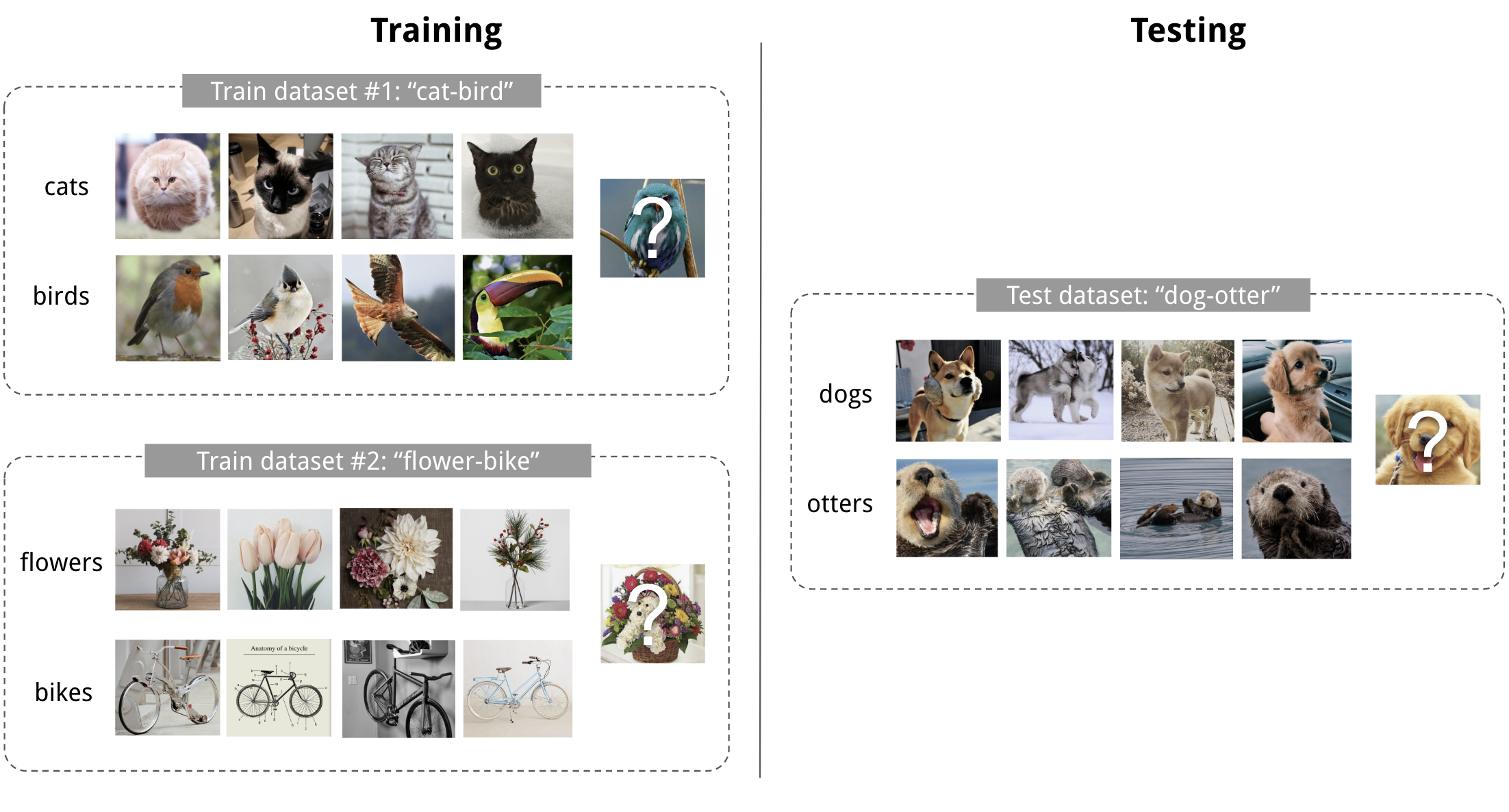Fig. 1. 4-shot 2-class 图像分类的例子。 (图像来自Pinterest)

### 像测试一样训练

\begin{aligned} \theta^* &= {\arg\max}_{\theta} \mathbb{E}_{(\mathbf{x}, y)\in \mathcal{D}}[P_\theta(y \vert \mathbf{x})] &\\ \theta^* &= {\arg\max}_{\theta} \mathbb{E}_{B\subset \mathcal{D}}[\sum_{(\mathbf{x}, y)\in B}P_\theta(y \vert \mathbf{x})] & \scriptstyle{\text{; trained with mini-batches.}} \end{aligned}

few-shot classification的目标是，在小规模的support set上“快速学习”（类似fine-tuning）后，能够减少在prediction set上的预测误差。为了训练模型快速学习的能力，我们在训练的时候按照以下步骤：

1. 采样一个标签的子集, $$L\subset\mathcal{L}$$ .
2. 根据采样的标签子集，采样一个support set $$S^L \subset \mathcal{D}$$ 和一个training batch $$B^L \subset \mathcal{D}$$ 。 $$S^L$$ 和 $$B^L$$ 中的数据的标签都属于 $$L$$ ，即 $$y \in L, \forall (x, y) \in S^L, B^L$$ .
3. 把support set作为模型的输入，进行“快速学习”。注意，不同的算法具有不同的学习策略，但总的来说，这一步不会永久性更新模型参数。
4. 把prediction set作为模型的输入，计算模型在 $$B^L$$ 上的loss，根据这个loss进行反向传播更新模型参数。这一步与监督学习一致。

$\theta = \arg\max_\theta \color{red}{E_{L\subset\mathcal{L}}[} E_{\color{red}{S^L \subset\mathcal{D}, }B^L \subset\mathcal{D}} [\sum_{(x, y)\in B^L} P_\theta(x, y\color{red}{, S^L})] \color{red}{]}$

### 学习器和元学习器

• 根据给定的任务，训练一个分类器 $$f_\theta$$ 完成任务，作为“学习器”模型
• 同时，训练一个元学习器 $$g_\phi$$ ，根据support set $$S$$ 学习如何更新学习器模型的参数。 $$\theta' = g_\phi(\theta, S)$$

$\mathbb{E}_{L\subset\mathcal{L}}[ \mathbb{E}_{S^L \subset\mathcal{D}, B^L \subset\mathcal{D}} [\sum_{(\mathbf{x}, y)\in B^L} P_{g_\phi(\theta, S^L)}(y \vert \mathbf{x})]]$

### 常见方法

Model-based Metric-based Optimization-based
Key idea RNN; memory Metric learning Gradient descent
How $$P_\theta(y \vert \mathbf{x})$$ is modeled? $$f_\theta(\mathbf{x}, S)$$ $$\sum_{(\mathbf{x}_i, y_i) \in S} k_\theta(\mathbf{x}, \mathbf{x}_i)y_i$$ (*) $$P_{g_\phi(\theta, S^L)}(y \vert \mathbf{x})$$

(*) $$k_\theta$$ 是一个衡量 $$\mathbf{x}_i$$ 和 $$\mathbf{x}$$ 相似度的kernel function。

## 基于度量的方法

$P_\theta(y \vert \mathbf{x}, S) = \sum_{(\mathbf{x}_i, y_i) \in S} k_\theta(\mathbf{x}, \mathbf{x}_i)y_i$

### Convolutional Siamese Neural Network

Siamese Neural Network最早被提出用来解决笔迹验证问题，siamese network由两个孪生网络组成，这两个网络的输出被联合起来训练一个函数，用于学习一对数据输入之间的关系。这两个网络结构相同，共享参数，实际上就是一个网络在学习如何有效地embedding才能显现出一对数据之间的关系。顺便一提，这是LeCun 1994年的论文。

Koch, Zemel & Salakhutdinov (2015)提出了一种用siamese网络做one-shot image classification的方法。首先，训练一个用于图片验证的siamese网络，分辨两张图片是否属于同一类。然后在测试时，siamese网络把测试输入和support set里面的所有图片进行比较，选择相似度最高的那张图片所属的类作为输出。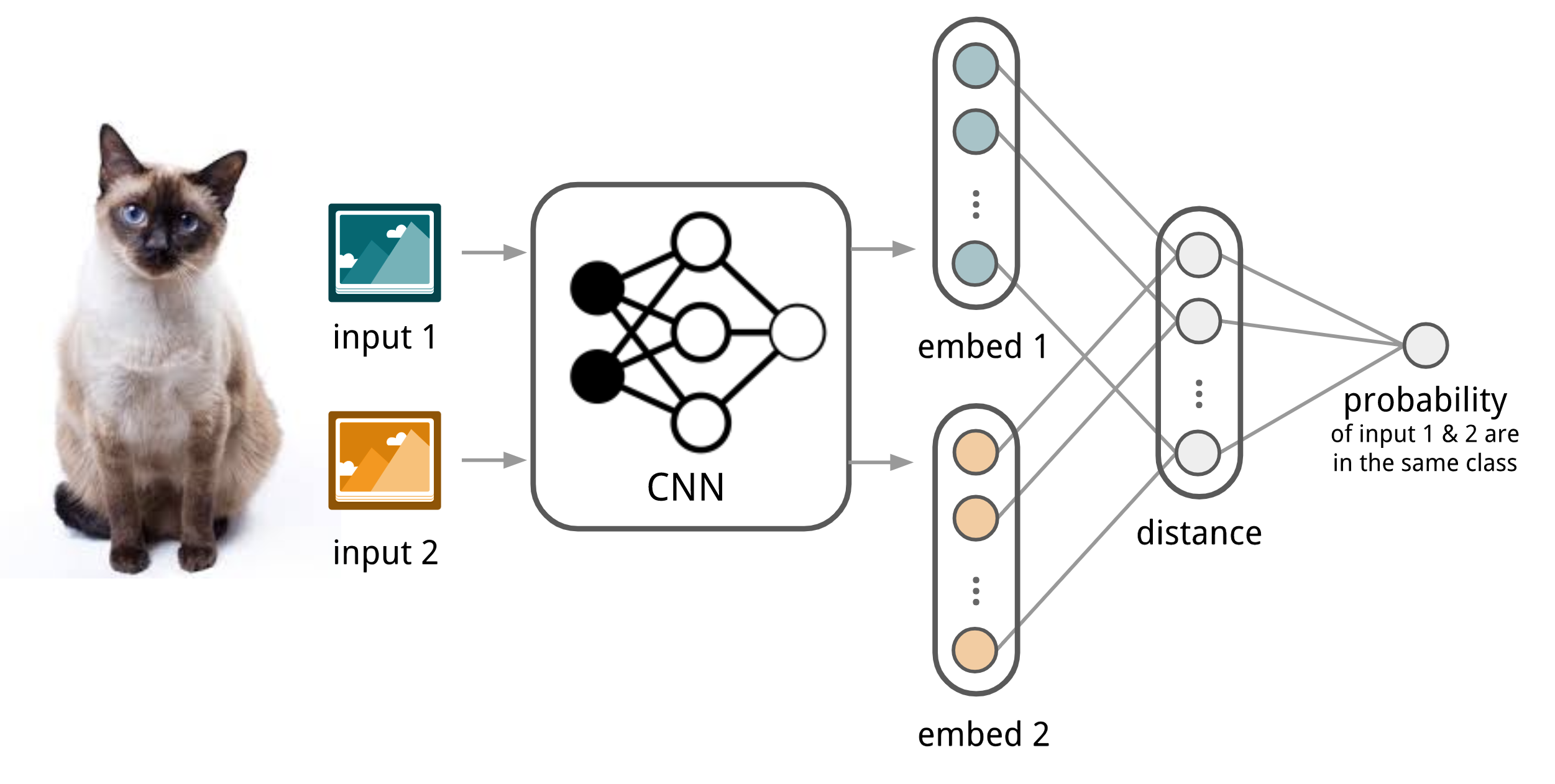Fig. 2. 卷积siamese网络用于few-shot image classification的例子。

1. 首先，卷积siamese网络学习一个由多个卷积层组成的embedding函数 $$f_\theta$$ ，把两张图片编码为特征向量。
2. 两个特征向量之间的L1距离可以表示为 $$\vert f_\theta(\mathbf{x}_i) - f_\theta(\mathbf{x}_j) \vert$$ 。
3. 通过一个linear feedforward layer和sigmoid把距离转换为概率。这就是两张图片属于同一类的概率。
4. loss函数就是cross entropy loss，因为label是二元的。
\begin{aligned} p(\mathbf{x}_i, \mathbf{x}_j) &= \sigma(\mathbf{W}\vert f_\theta(\mathbf{x}_i) - f_\theta(\mathbf{x}_j) \vert) \\ \mathcal{L}(B) &= \sum_{(\mathbf{x}_i, \mathbf{x}_j, y_i, y_j)\in B} \mathbf{1}_{y_i=y_j}\log p(\mathbf{x}_i, \mathbf{x}_j) + (1-\mathbf{1}_{y_i=y_j})\log (1-p(\mathbf{x}_i, \mathbf{x}_j)) \end{aligned}

Training batch $$B$$ 可以通过对图片做一些变形增加数据量。你也可以把L1距离替换成其他距离，比如L2距离、cosine距离等等。只要距离是可导的就可以。

$\hat{c}_S(\mathbf{x}) = c(\arg\max_{\mathbf{x}_i \in S} P(\mathbf{x}, \mathbf{x}_i))$

$$c(\mathbf{x})$$ 是图片 $$\mathbf{x}$$ 的label， $$\hat{c}(.)$$ 是预测的label。

### Matching Networks

Matching Networks (Vinyals et al., 2016)的目标是：对于每一个给定的支持集 $$S=\{x_i, y_i\}_{i=1}^k$$ (k-shot classification)，分别学一个分类器 $$c_S$$ 。 这个分类器给出了给定测试样本 $$\mathbf{x}$$ 时，输出 $$y$$ 的概率分布。这个分类器的输出被定义为支持集中一系列label的加权和，权重由一个注意力核（attention kernel） $$a(\mathbf{x}, \mathbf{x}_i)$$ 决定。权重应当与 $$\mathbf{x}$$ 和 $$\mathbf{x}_i$$ 间的相似度成正比。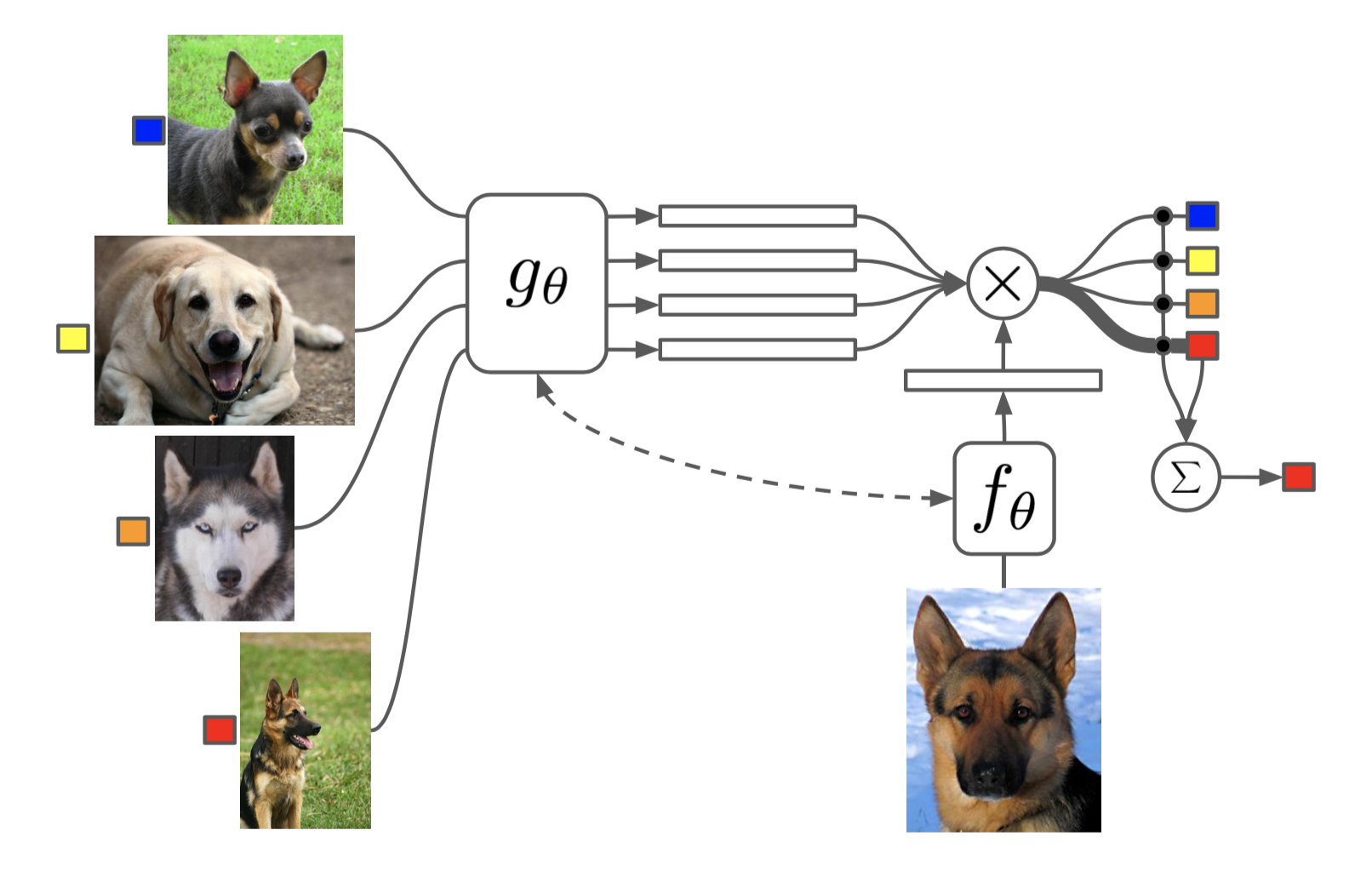Fig. 3. Matching Networks结构。（图像来源: 原论文

$c_S(\mathbf{x}) = P(y \vert \mathbf{x}, S) = \sum_{i=1}^k a(\mathbf{x}, \mathbf{x}_i) y_i \text{, where }S=\{(\mathbf{x}_i, y_i)\}_{i=1}^k$

Attention kernel由两个embedding function $$f$$ 和 $$g$$ 决定。分别用于encoding测试样例和支持集样本。两个样本之间的注意力权重是经过softmax归一化后的，他们embedding vectors的cosine距离 $$\text{cosine}(.)$$ 。

$a(\mathbf{x}, \mathbf{x}_i) = \frac{\exp(\text{cosine}(f(\mathbf{x}), g(\mathbf{x}_i))}{\sum_{j=1}^k\exp(\text{cosine}(f(\mathbf{x}), g(\mathbf{x}_j))}$

#### Full Context Embeddings

Embeding vectors对于构建一个好的分类器至关重要。只把一个数据样本作为embedding function的输入，会导致很难高效的估计出整个特征空间。因此，Matching Network模型又进一步发展，通过把整个支持集 $$S$$ 作为embedding function的额外输入来加强embedding的有效性，相当于给样本添加了语境，让embedding根据样本与支持集中样本的关系进行调整。

• $$g_\theta(\mathbf{x}_i, S)$$ 在整个支持集 $$S$$ 的语境下用一个双向LSTM来编码 $$\mathbf{x}_i$$ .

• $$f_\theta(\mathbf{x}, S)$$ 在支持集 $$S$$ 上使用read attention机制编码测试样本 $$\mathbf{x}$$ 。

1. 首先测试样本经过一个简单的神经网络，比如CNN，以抽取基本特征 $$f'(\mathbf{x})$$ 。
\begin{aligned} \hat{\mathbf{h}}_t, \mathbf{c}_t &= \text{LSTM}(f'(\mathbf{x}), [\mathbf{h}_{t-1}, \mathbf{r}_{t-1}], \mathbf{c}_{t-1}) \\ \mathbf{h}_t &= \hat{\mathbf{h}}_t + f'(\mathbf{x}) \\ \mathbf{r}_{t-1} &= \sum_{i=1}^k a(\mathbf{h}_{t-1}, g(\mathbf{x}_i)) g(\mathbf{x}_i) \\ a(\mathbf{h}_{t-1}, g(\mathbf{x}_i)) &= \text{softmax}(\mathbf{h}_{t-1}^\top g(\mathbf{x}_i)) = \frac{\exp(\mathbf{h}_{t-1}^\top g(\mathbf{x}_i))}{\sum_{j=1}^k \exp(\mathbf{h}_{t-1}^\top g(\mathbf{x}_j))} \end{aligned}
3. 最终，如果我们做k步的读取 $$f(\mathbf{x}, S)=\mathbf{h}_K$$ 。

Matching Networks的训练过程与测试时的推理过程是一致的，详情请回顾之前的章节。值得一提的是，Matching Networks的论文强调了训练和测试的条件应当一致的原则。

$\theta^* = \arg\max_\theta \mathbb{E}_{L\subset\mathcal{L}}[ \mathbb{E}_{S^L \subset\mathcal{D}, B^L \subset\mathcal{D}} [\sum_{(\mathbf{x}, y)\in B^L} P_\theta(y\vert\mathbf{x}, S^L)]]$

### Relation Network

Relation Network (RN) (Sung et al., 2018)与siamese network比较像，但有以下几个不同点：

1. 两个样本间的相似系数不是由特征空间的L1距离决定的，而是由一个CNN分类器 $$g_\phi$$ 预测的。两个样本 $$\mathbf{x}_i$$ 和 $$\mathbf{x}_j$$ 间的相似系数为 $$r_{ij} = g_\phi([\mathbf{x}_i, \mathbf{x}_j])$$ ，其中 $$[.,.]$$ 代表着concatenation。
2. 目标优化函数是MSE损失，而不是cross-entropy，因为RN在预测时更倾向于把相似系数预测过程作为一个regression问题，而不是二分类问题， $$\mathcal{L}(B) = \sum_{(\mathbf{x}_i, \mathbf{x}_j, y_i, y_j)\in B} (r_{ij} - \mathbf{1}_{y_i=y_j})^2$$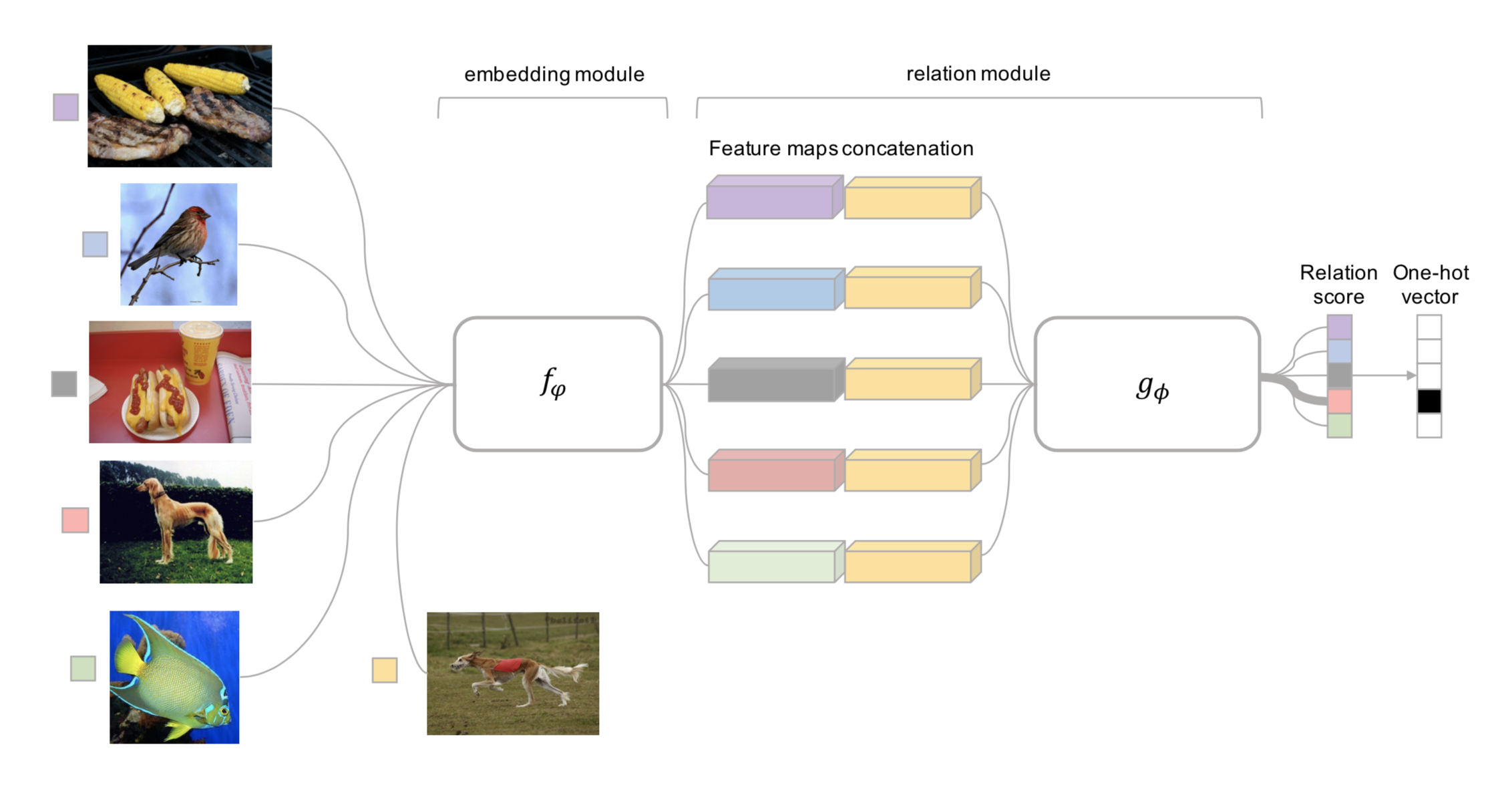Fig. 4. Relation Network的结构，图中是一个5分类1-shot的例子。(图片来源：原论文)

(注意：还有一个Relation Network是DeepMind提出来用于关系推理的，不要搞混了。)

### Prototypical Networks

Prototypical Networks (Snell, Swersky & Zemel, 2017)用一个嵌入函数 $$f_\theta$$ 把每个输入编码为一个M维特征向量。然后对每一类 $$c \in \mathcal{C}$$ ，取所有支持集样本的特征向量的平均值作为这个类的prototype特征。

$\mathbf{v}_c = \frac{1}{|S_c|} \sum_{(\mathbf{x}_i, y_i) \in S_c} f_\theta(\mathbf{x}_i)$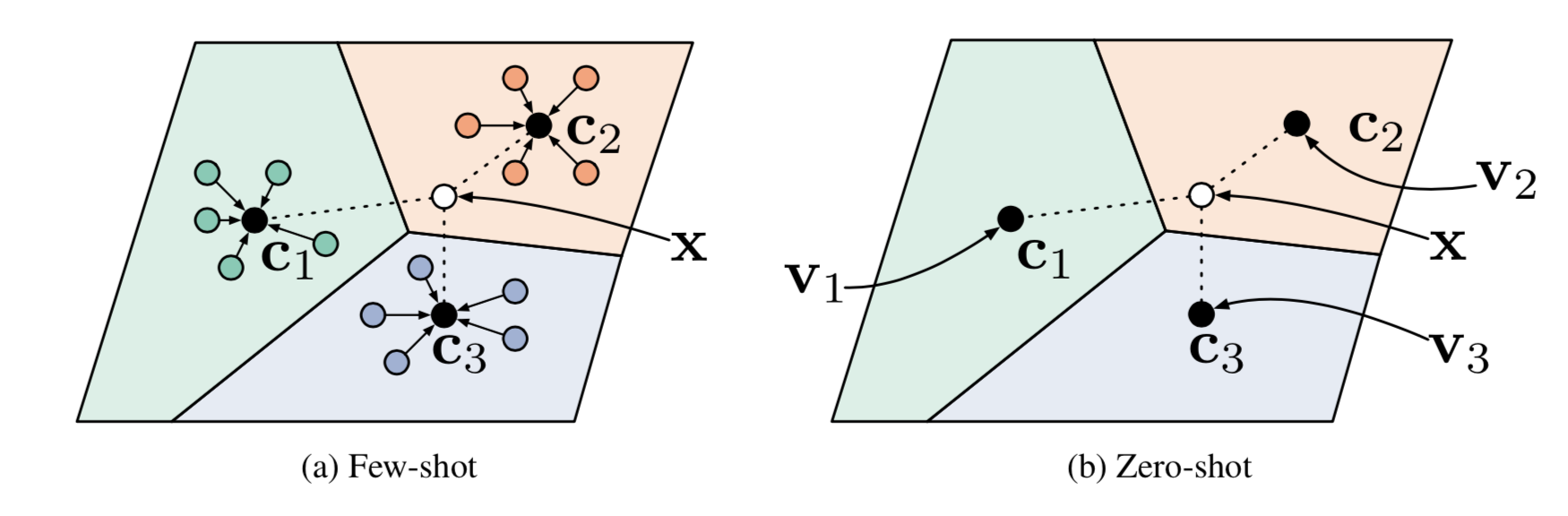Fig. 5. 在少样本学习和无样本学习中的Prototypical networks。(图像来源：原论文)

$P(y=c\vert\mathbf{x})=\text{softmax}(-d_\varphi(f_\theta(\mathbf{x}), \mathbf{v}_c)) = \frac{\exp(-d_\varphi(f_\theta(\mathbf{x}), \mathbf{v}_c))}{\sum_{c' \in \mathcal{C}}\exp(-d_\varphi(f_\theta(\mathbf{x}), \mathbf{v}_{c'}))}$

## 基于模型的方法

### Memory-Augmented Neural Networks

MANN的目的是在仅给定几个训练样本的情况下，快速编码新的信息并适应新的任务，因此MANN非常适合用于元学习。Santoro et al. (2016)以Neural Turing Machine（NTM）为基础，对训练和存储读写机制（也称为“寻址机制”，即如何对存储向量分配注意力权重）做了一系列的修改。如果你对NTM或寻址机制不太熟悉的话，可以参见原作者之前博文中的NTM介绍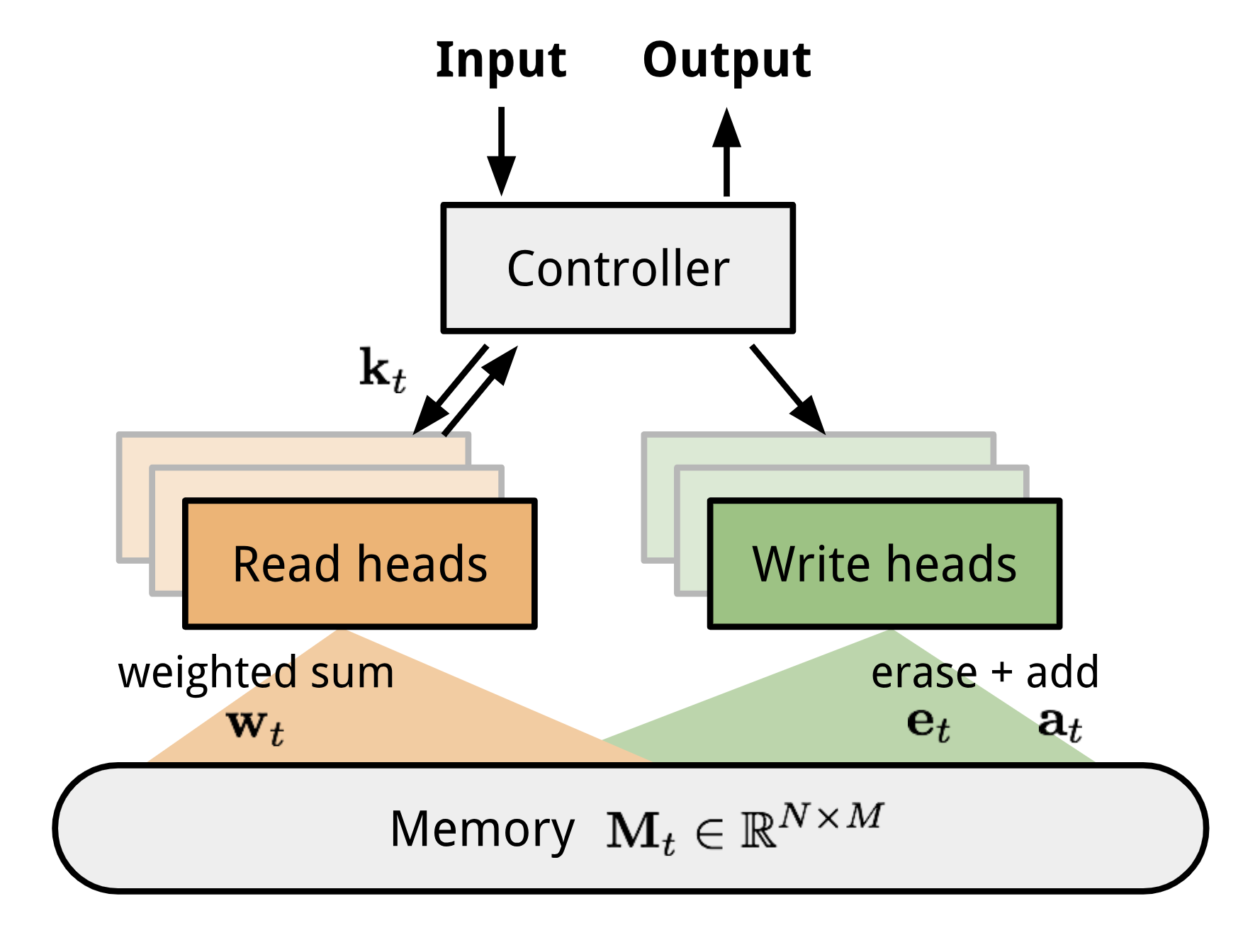Fig. 6. NTM的架构，t时刻的存储， $$\mathbf{M}_t$$ 是一个大小为 $$N \times M$$ 的矩阵，代表着N个M维的向量，每个向量是一条记录

#### MANN for Meta-Learning

Santoro et al., 2016提出了一种有意思的训练方式，他们强迫存储器保留当前样本的信息直到对应的标签出现。在每个episode中，标签有 $$一步的延迟$$ ，即每次给出的训练对为 $$(\mathbf{x}_{t+1}, y_t)$$ 。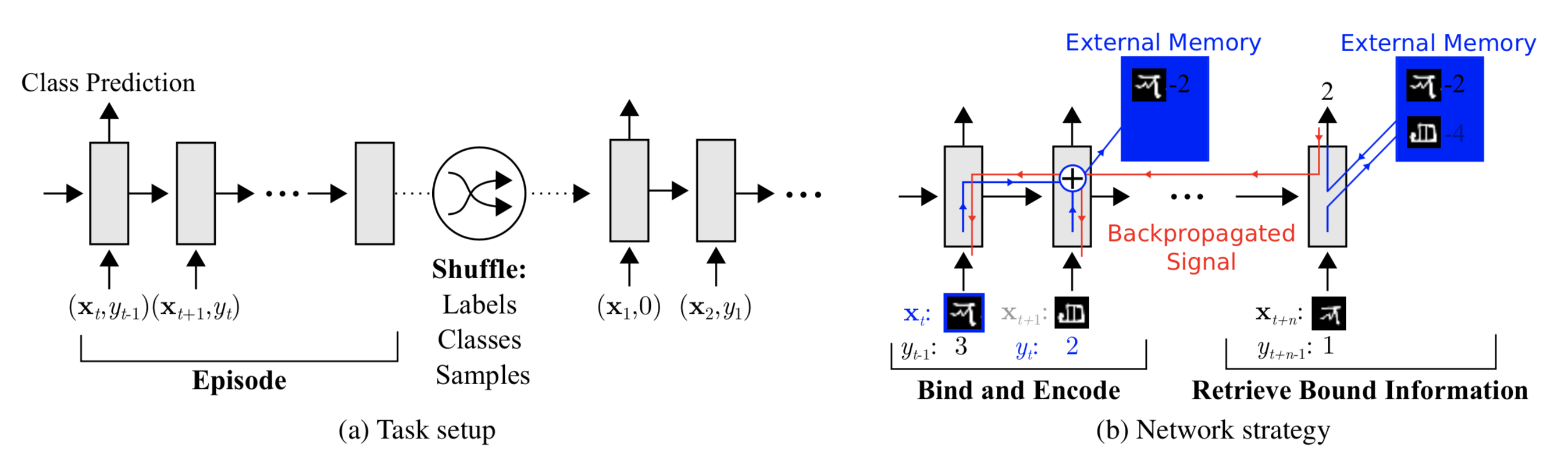Fig. 7. MANN在元学习中的任务设置（图像来源：原论文）。

» 如何从存储器中取回信息?

$\mathbf{r}_i = \sum_{i=1}^N w_t^r(i)\mathbf{M}_t(i) \text{, where } w_t^r(i) = \text{softmax}(\frac{\mathbf{k}_t \cdot \mathbf{M}_t(i)}{\|\mathbf{k}_t\| \cdot \|\mathbf{M}_t(i)\|})$

$$M_t$$ 是t时刻的存储器矩阵， $$M_t(i)$$ 是该矩阵中的第i行，即第i个向量。

» 如何往存储器中写入信息?

• 最少使用的位置：目的是保存经常使用的那些信息(参见LFU);
• 最近使用的位置：原因是刚用过的信息很有可能不会马上用到(参见MRU).

1. t时刻的使用权重 $$\mathbf{w}^u_t$$ 是当前读写向量的和，再加上上一时刻的使用权重 $$\gamma \mathbf{w}^u_{t-1}$$ ， $$\gamma$$ 是一个衰减系数。
2. 写入向量由之前的读取权重（代表着最近使用的位置）和之前的最少使用权重（代表着最少使用的位置）插值得到。插值参数是超参数 $$\alpha$$ 的sigmoid。
3. 最少使用权重 $$\mathbf{w}_t^{lu}$$ 由使用权重 $$\mathbf{w}_t^u$$ 二值化得到。首先选取 $$\mathbf{w}_t^u$$ 第n小的元素，找出 $$\mathbf{w}_t^u$$ 中所有比这个元素小的元素，对应的位置在 $$\mathbf{w}_t^{lu}$$ 中设为1，反之则设为0。
\begin{aligned} \mathbf{w}_t^u &= \gamma \mathbf{w}_{t-1}^u + \mathbf{w}_t^r + \mathbf{w}_t^w \\ \mathbf{w}_t^r &= \text{softmax}(\text{cosine}(\mathbf{k}_t, \mathbf{M}_t(i))) \\ \mathbf{w}_t^w &= \sigma(\alpha)\mathbf{w}_{t-1}^r + (1-\sigma(\alpha))\mathbf{w}^{lu}_{t-1}\\ \mathbf{w}_t^{lu} &= \mathbf{1}_{w_t^u(i) \leq m(\mathbf{w}_t^u, n)} \text{, where }m(\mathbf{w}_t^u, n)\text{ is the }n\text{-th smallest element in vector }\mathbf{w}_t^u\text{.} \end{aligned}

### Meta Networks

Meta Networks (Munkhdalai & Yu, 2017)，简称MetaNet，是一个专门针对多任务间快速泛化设计的元学习模型，模型结构和训练过程都经过了调整。

#### Fast Weights

MetaNet的快速泛化能力依赖于“快参数（fast weights）”。有许多论文涉及这个话题，但我还没把他们全都仔细读过，没能找到一个具体的定义，只有一些模糊的共识。一般神经网络的权重是根据目标函数进行随机梯度下降更新的，但这个过程很慢。一种更快的学习方法是利用另外一个神经网络，预测当前神经网络的参数，预测出来的参数被称为快参数。而普通SGD生成的权重则被称为慢参数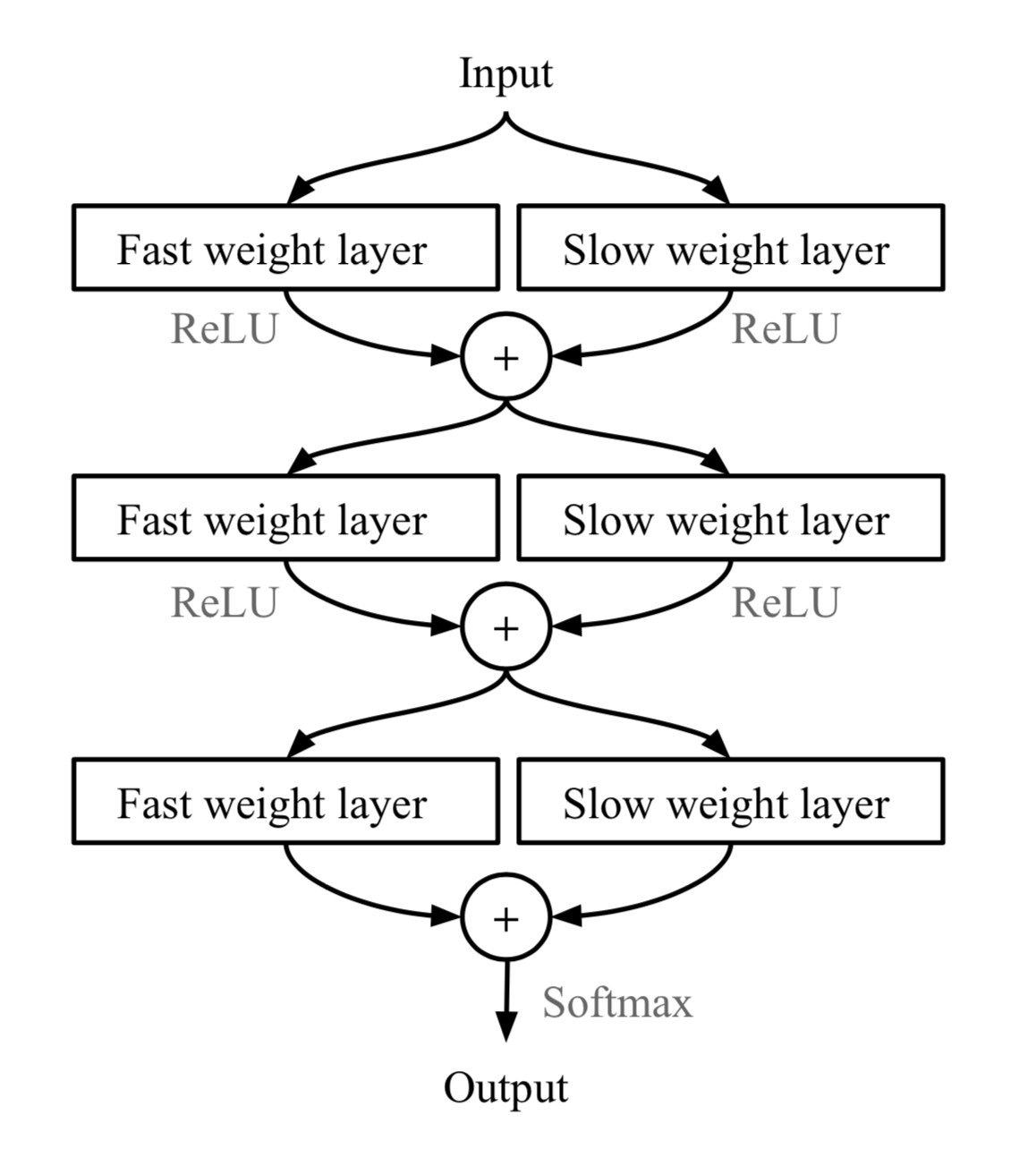Fig. 8. 结合了慢参数和快参数的MLP。 $$\bigoplus$$ is element-wise sum. (图像来源：原论文).

#### Model Components

MetaNet的关键组件是：

• $$f_\theta$$ ：一个由 $$\theta$$ 决定的编码函数，发挥着元学习器的作用。负责把原始输入编码为特征向量。类似Siamese Neural Network，我们希望训练这个编码函数使得能够根据其生成的特征向量判断两个输入是否属于同一类（验证任务）。
• $$g_\phi$$ ：一个由 $$\phi$$ 决定的基学习器，负责完成真正的学习任务。

• $$F_w$$ ：一个由 $$w$$ 决定的LSTM，用于学习嵌入函数 $$f$$ 的快参数 $$\theta^+$$ 。它把 $$f$$ 在验证任务上的loss梯度作为输入。
• $$G_v$$ ：一个由 $$v$$ 决定的神经网络，根据基学习器 $$g$$ 的loss梯度学习其快参数 $$\phi^+$$ 。在MetaNet中，学习器的loss梯度被视作任务的元信息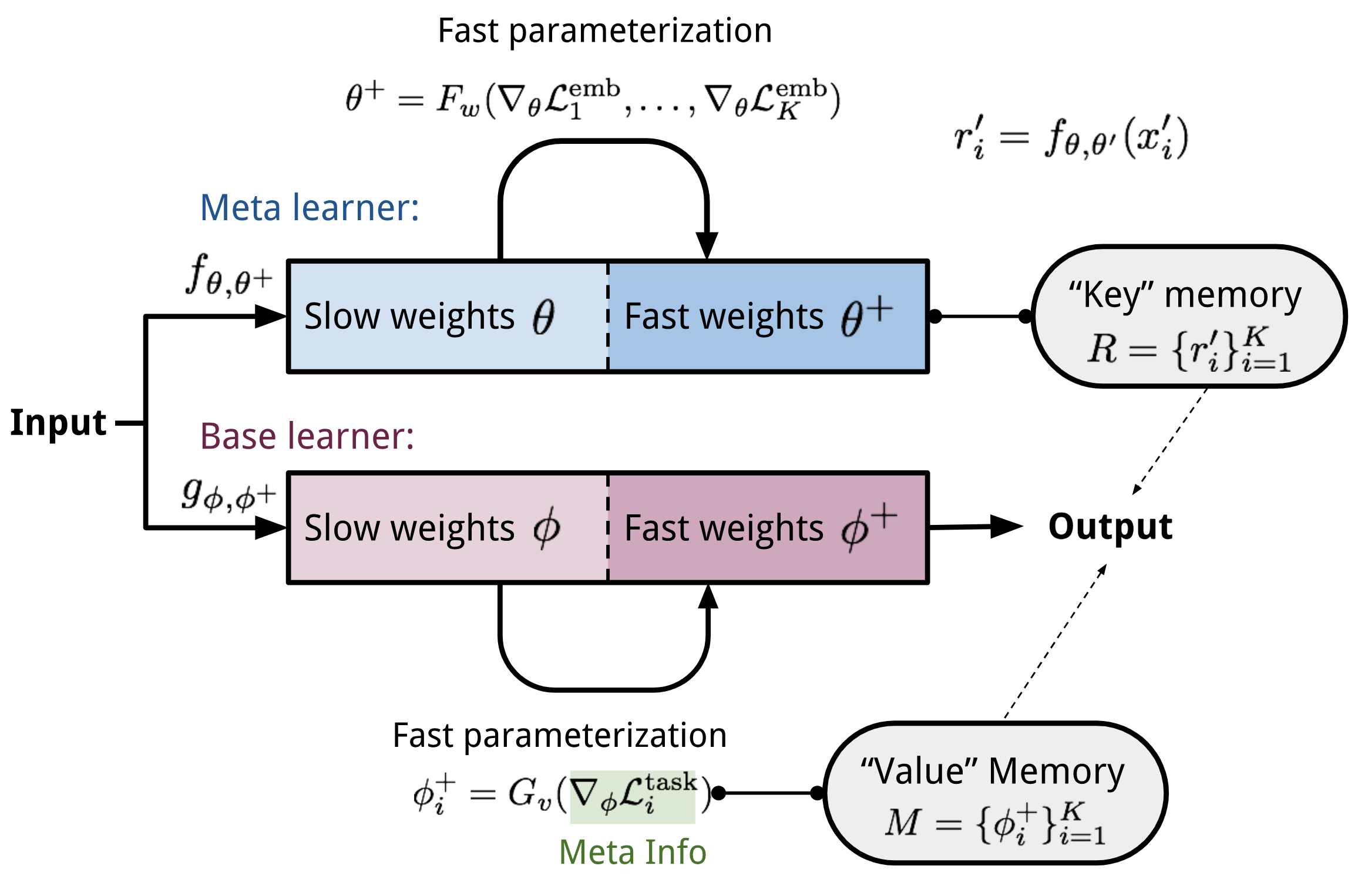Fig.9. MetaNet的结构。

#### 训练过程

1. 从支持集 $$S$$ 中随机采样一对输入， $$(\mathbf{x}'_i, y'_i)$$ 和 $$(\mathbf{x}'_j, y_j)$$ 。令 $$\mathbf{x}_{(t,1)}=\mathbf{x}'_i$$ ， $$\mathbf{x}_{(t,2)}=\mathbf{x}'_j$$ .
for $$t = 1, \dots, K$$ :
• a. 计算编码函数的loss，即验证任务上的交叉熵
$$\mathcal{L}^\text{emb}_t = \mathbf{1}_{y'_i=y'_j} \log P_t + (1 - \mathbf{1}_{y'_i=y'_j})\log(1 - P_t)\text{, where }P_t = \sigma(\mathbf{W}\vert f_\theta(\mathbf{x}_{(t,1)}) - f_\theta(\mathbf{x}_{(t,2)})\vert)$$
2. 根据编码函数的loss计算任务级别的快参数: $$\theta^+ = F_w(\nabla_\theta \mathcal{L}^\text{emb}_1, \dots, \mathcal{L}^\text{emb}_T)$$
3. 接下来遍历支持集 $$S$$ 中的样本，计算样本级别的快参数
for $$i=1, \dots, K$$ :
• a. 基学习器输出一个概率分布： $$P(\hat{y}_i \vert \mathbf{x}_i) = g_\phi(\mathbf{x}_i)$$ ，loss可以用交叉熵或者MSE: $$\mathcal{L}^\text{task}_i = y'_i \log g_\phi(\mathbf{x}'_i) + (1- y'_i) \log (1 - g_\phi(\mathbf{x}'_i))$$
• b. 提取任务的元信息（loss的梯度）并计算样本级别的快参数: $$\phi_i^+ = G_v(\nabla_\phi\mathcal{L}^\text{task}_i)$$
• 把 $$\phi^+_i$$ 放在“值”存储器 $$\mathbf{M}$$ 的第 $$i$$ 个位置。
• d. 编码器结合慢参数和快参数对支持集中的样本进行编码： $$r'_i = f_{\theta, \theta^+}(\mathbf{x}'_i)$$
• 把 $$r'_i$$ 放在“键”存储器 $$\mathbf{R}$$ 的第 $$i$$ 个位置。
4. 最后就是要来根据测试集 $$U=\{\mathbf{x}_i, y_i\}_{i=1}^L$$ 来构建训练的loss了。注意，在这一步中，我们不使用 $$F_w$$ 和 $$G_v$$ 计算快参数。编码器的快参数保持不变，而基学习器的快参数则根据查询存储器得到。
从 $$\mathcal{L}_\text{train}=0$$ 开始：
for $$j=1, \dots, L$$ :
• a. 使用编码器结合慢参数和之前得到的快参数对测试样例进行编码： $$r_j = f_{\theta, \theta^+}(\mathbf{x}_j)$$
• b. 运用注意力机制，查找编码得到的 $$r_j$$ 在“键”存储器 $$\mathbf{R}$$ 的位置，然后拿出对应位置“值”存储器 $$\mathbf{M}$$ 对应位置的快参数，组合成当前测试样本对应的基学习器快参数。注意力函数可以随便选，MetaNet用的是cosine距离
\begin{aligned} a_j &= \text{cosine}(\mathbf{R}, r_j) = [\frac{r'_1\cdot r_j}{\|r'_1\|\cdot\|r_j\|}, \dots, \frac{r'_N\cdot r_j}{\|r'_N\|\cdot\|r_j\|}]\\ \phi^+_j &= \text{softmax}(a_j)^\top \mathbf{M} \end{aligned}
• c. 更新训练loss： $$\mathcal{L}_\text{train} \leftarrow \mathcal{L}_\text{train} + \mathcal{L}^\text{task}(g_{\phi, \phi^+}(\mathbf{x}_i), y_i)$$
5. 根据 $$\mathcal{L}_\text{train}$$ 更新所有参数 $$(\theta, \phi, w, v)$$ .

## 基于优化的方法

### LSTM Meta-Learner

Ravi & Larochelle (2017) 把优化算法显式的建模出来，并命名为“元学习器”，原本处理任务的模型被称为“学习器”。元学习器的目标是使用少量支持集在仅仅几步之内快速更新学习器的参数，使得学习器能够快速适应新任务。

#### 为什么使用 LSTM？

1. 反向传播中基于梯度的更新跟 LSTM 中 cell 状态的更新有相似之处。
2. 知道之前的梯度对当前的梯度更新有好处。可以参考 momentum 的原理。

$\theta_t = \theta_{t-1} - \alpha_t \nabla_{\theta_{t-1}}\mathcal{L}_t$

\begin{aligned} c_t &= f_t \odot c_{t-1} + i_t \odot \tilde{c}_t\\ &= \theta_{t-1} - \alpha_t\nabla_{\theta_{t-1}}\mathcal{L}_t \end{aligned}

\begin{aligned} f_t &= \sigma(\mathbf{W}_f \cdot [\nabla_{\theta_{t-1}}\mathcal{L}_t, \mathcal{L}_t, \theta_{t-1}, f_{t-1}] + \mathbf{b}_f) & \scriptstyle{\text{; how much to forget the old value of parameters.}}\\ i_t &= \sigma(\mathbf{W}_i \cdot [\nabla_{\theta_{t-1}}\mathcal{L}_t, \mathcal{L}_t, \theta_{t-1}, i_{t-1}] + \mathbf{b}_i) & \scriptstyle{\text{; corresponding to the learning rate at time step t.}}\\ \tilde{\theta}_t &= -\nabla_{\theta_{t-1}}\mathcal{L}_t &\\ \theta_t &= f_t \odot \theta_{t-1} + i_t \odot \tilde{\theta}_t &\\ \end{aligned}

#### Model Setup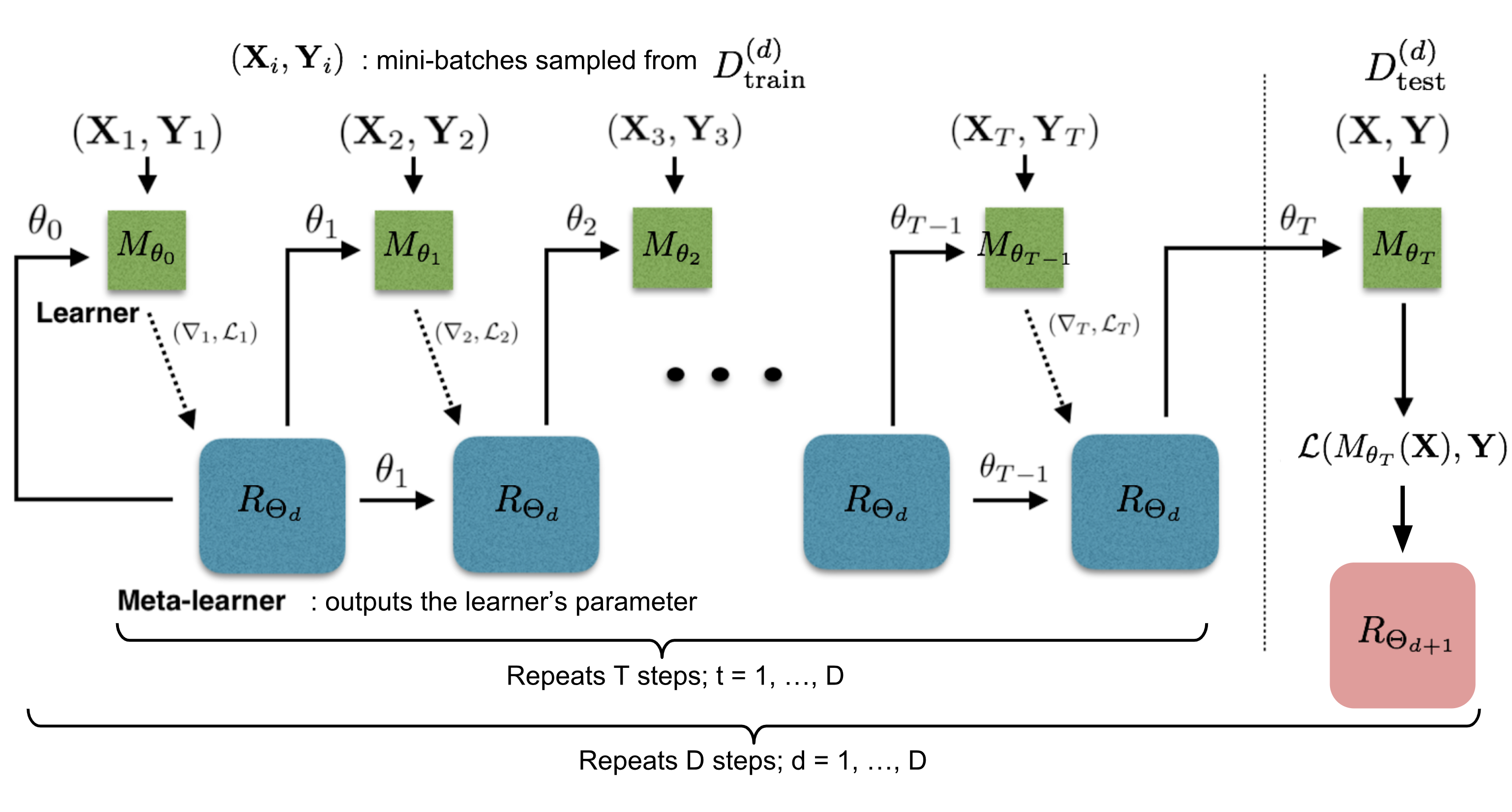Fig.10. 如何训练学习器 $$M_\theta$$ 和元学习器 $$R_\Theta$$. (图像来源：原论文中的图片加了一些注释)

Matching Networks中我们已经证明了用模仿测试过程的方式训练能够取得很好的效果，这里也用了类似的方法。在每个训练阶段，我们先采样一个数据集 $$\mathcal{D} = (\mathcal{D}_\text{train}, \mathcal{D}_\text{test}) \in \hat{\mathcal{D}}_\text{meta-train}$$，再从 $$\mathcal{D}_\text{train}$$ 中采样 $$T$$ 轮 mini-batches 用于更新 $$\theta$$。学习器参数的最终状态$$\theta_T$$被用来在测试数据 $$\mathcal{D}_\text{test}$$ 上训练元学习器。

1. 如何压缩 LSTM 元学习的参数空间？元学习器是在建模一个神经网络的参数，所以有上百万个变量要学。为了减小元学习器的参数空间，这篇文章借鉴了共享参数的方法。元学习器本质上学习的是一种更新原则，即如何根据一个参数的值和其梯度生成这个参数的新值（比如一阶方法，牛顿法等），与参数在学习器中的位置无关。所以我们可以假设所有参数的更新原则都是一样的，即元学习只需要输出一维变量即可。
2. 为了简化训练过程，元学习器假设 loss $$\mathcal{L}_t$$ 和梯度 $$\nabla_{\theta_{t-1}} \mathcal{L}_t$$ 是独立的。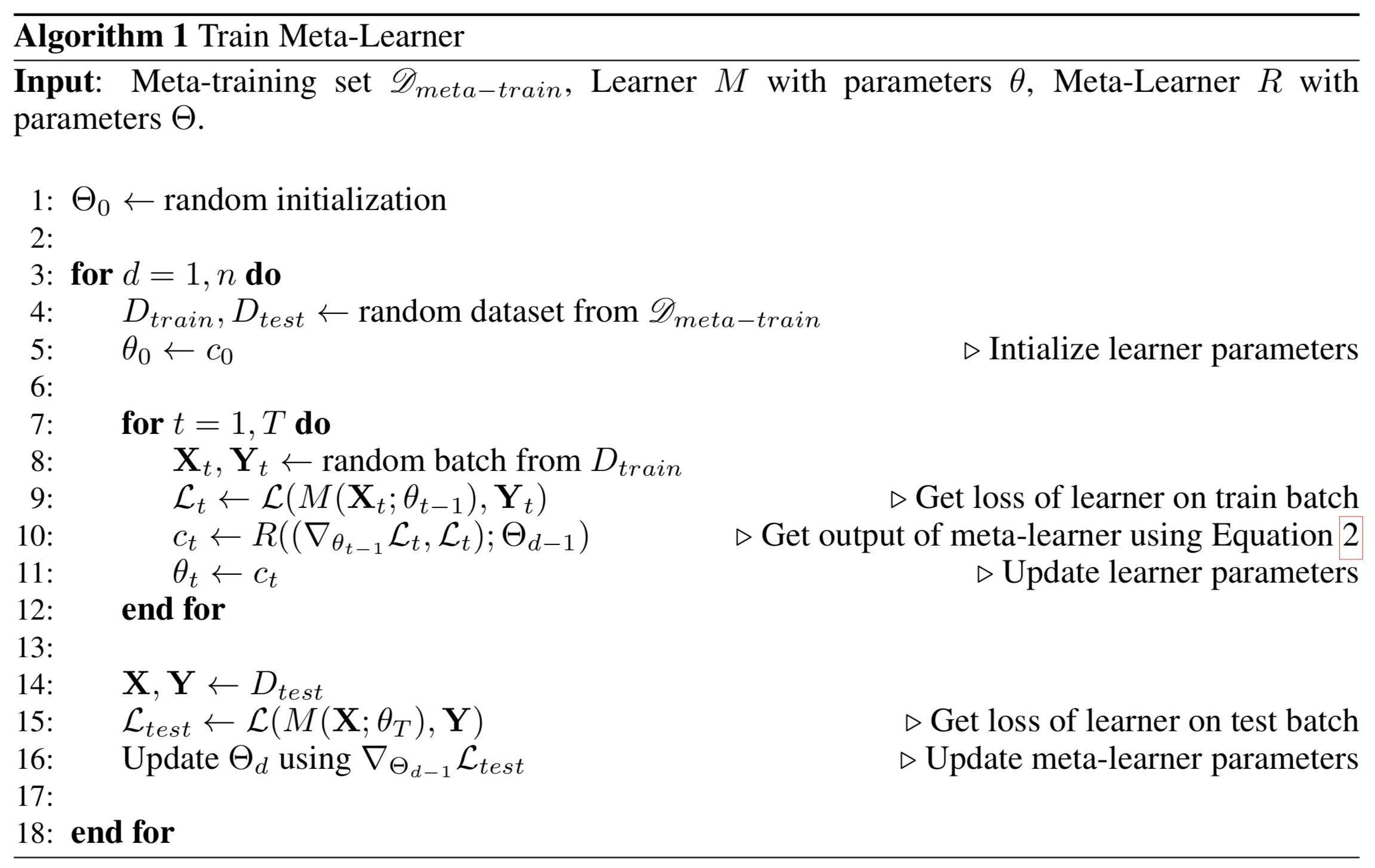### MAML

Model-Agnostic Meta-Learning 简称 MAML (Finn, et al. 2017)， 是一种非常通用的优化算法，可以被用于任何基于梯度下降学习的模型。

$\theta'_i = \theta - \alpha \nabla_\theta\mathcal{L}^{(0)}_{\tau_i}(f_\theta)$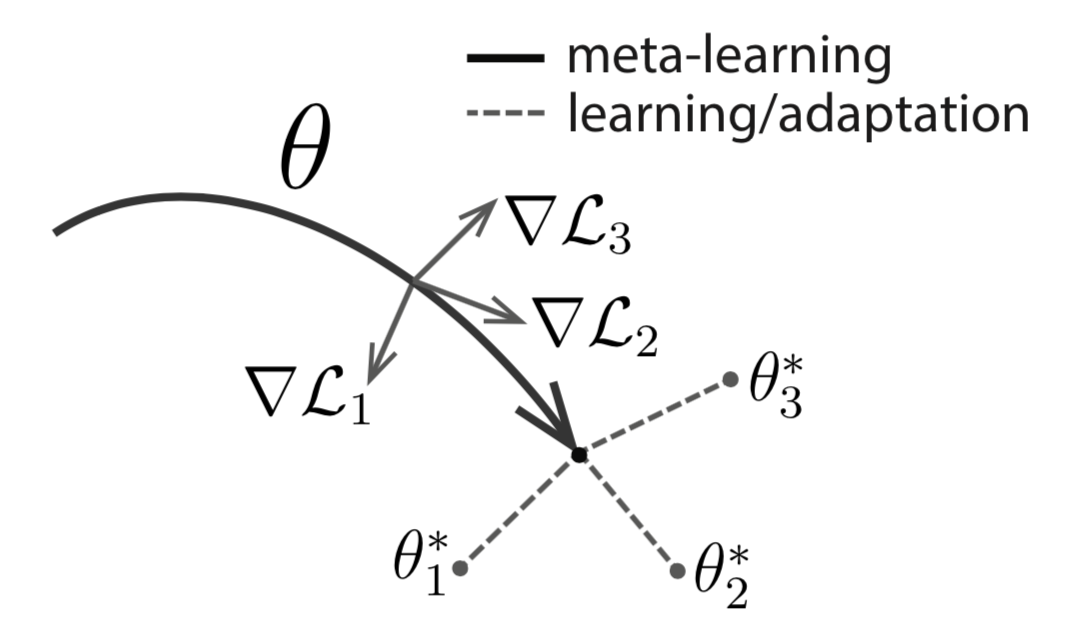Fig. 11. MAML图示。 (图像来源：原论文)

\begin{aligned} \theta^* &= \arg\min_\theta \sum_{\tau_i \sim p(\tau)} \mathcal{L}_{\tau_i}^{(1)} (f_{\theta'_i}) = \arg\min_\theta \sum_{\tau_i \sim p(\tau)} \mathcal{L}_{\tau_i}^{(1)} (f_{\theta - \alpha\nabla_\theta \mathcal{L}_{\tau_i}^{(0)}(f_\theta)}) & \\ \theta &\leftarrow \theta - \beta \nabla_{\theta} \sum_{\tau_i \sim p(\tau)} \mathcal{L}_{\tau_i}^{(1)} (f_{\theta - \alpha\nabla_\theta \mathcal{L}_{\tau_i}^{(0)}(f_\theta)}) & \scriptstyle{\text{; updating rule}} \end{aligned}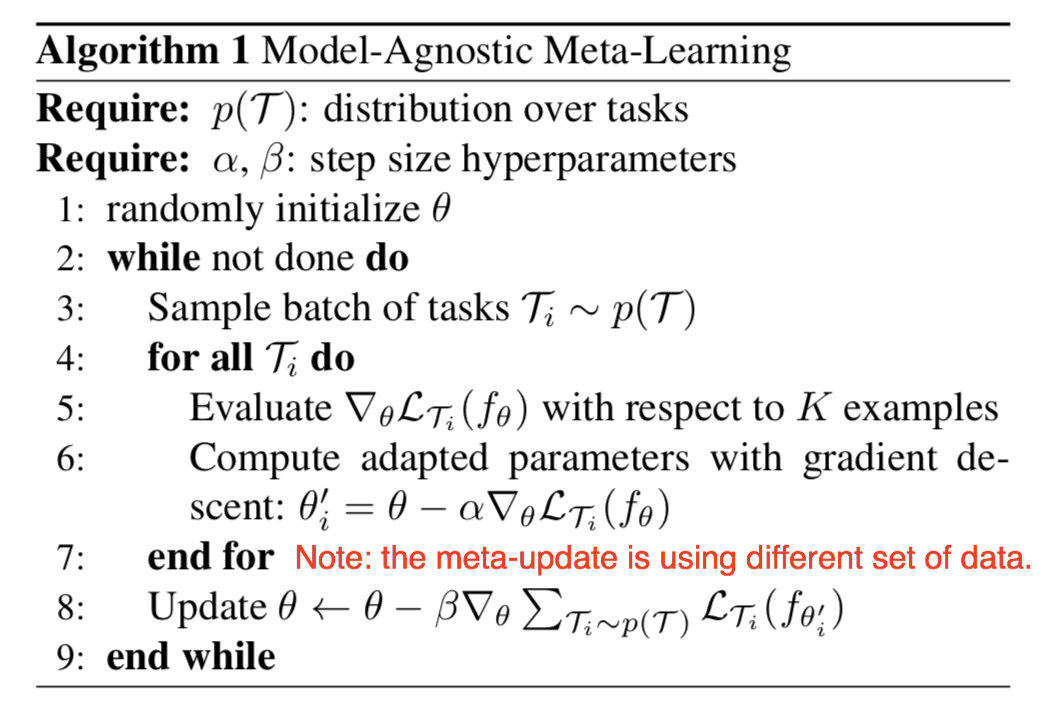Fig. 12. MAML算法的一般形式。 (图像来源：原论文)

#### First-Order MAML

\begin{aligned} \theta_0 &= \theta_\text{meta}\\ \theta_1 &= \theta_0 - \alpha\nabla_\theta\mathcal{L}^{(0)}(\theta_0)\\ \theta_2 &= \theta_1 - \alpha\nabla_\theta\mathcal{L}^{(0)}(\theta_1)\\ &\dots\\ \theta_k &= \theta_{k-1} - \alpha\nabla_\theta\mathcal{L}^{(0)}(\theta_{k-1}) \end{aligned}

\begin{aligned} \theta_\text{meta} &\leftarrow \theta_\text{meta} - \beta g_\text{MAML} & \scriptstyle{\text{; update for meta-objective}} \\[2mm] \text{其中 } g_\text{MAML} &= \nabla_{\theta} \mathcal{L}^{(1)}(\theta_k) &\\[2mm] &= \nabla_{\theta_k} \mathcal{L}^{(1)}(\theta_k) \cdot (\nabla_{\theta_{k-1}} \theta_k) \dots (\nabla_{\theta_0} \theta_1) \cdot (\nabla_{\theta} \theta_0) & \scriptstyle{\text{; following the chain rule}} \\ &= \nabla_{\theta_k} \mathcal{L}^{(1)}(\theta_k) \cdot \prod_{i=1}^k \nabla_{\theta_{i-1}} \theta_i & \\ &= \nabla_{\theta_k} \mathcal{L}^{(1)}(\theta_k) \cdot \prod_{i=1}^k \nabla_{\theta_{i-1}} (\theta_{i-1} - \alpha\nabla_\theta\mathcal{L}^{(0)}(\theta_{i-1})) & \\ &= \nabla_{\theta_k} \mathcal{L}^{(1)}(\theta_k) \cdot \prod_{i=1}^k (I - \alpha\nabla_{\theta_{i-1}}(\nabla_\theta\mathcal{L}^{(0)}(\theta_{i-1}))) & \end{aligned}

MAML的梯度是：

$g_\text{MAML} = \nabla_{\theta_k} \mathcal{L}^{(1)}(\theta_k) \cdot \prod_{i=1}^k (I - \alpha \color{red}{\nabla_{\theta_{i-1}}(\nabla_\theta\mathcal{L}^{(0)}(\theta_{i-1}))})$

$g_\text{FOMAML} = \nabla_{\theta_k} \mathcal{L}^{(1)}(\theta_k)$

### Reptile

Reptile (Nichol, Achiam & Schulman, 2018) 是一个超级简单的元学习优化算法。它跟 MAML 类似，它们都靠梯度下降进行元优化，而且都是模型无关的算法。

Reptiled 的执行流程如下:

• 1) 采样一个任务,
• 2) 在这个任务上进行多次梯度下降
• 3) 把模型参数向新参数靠近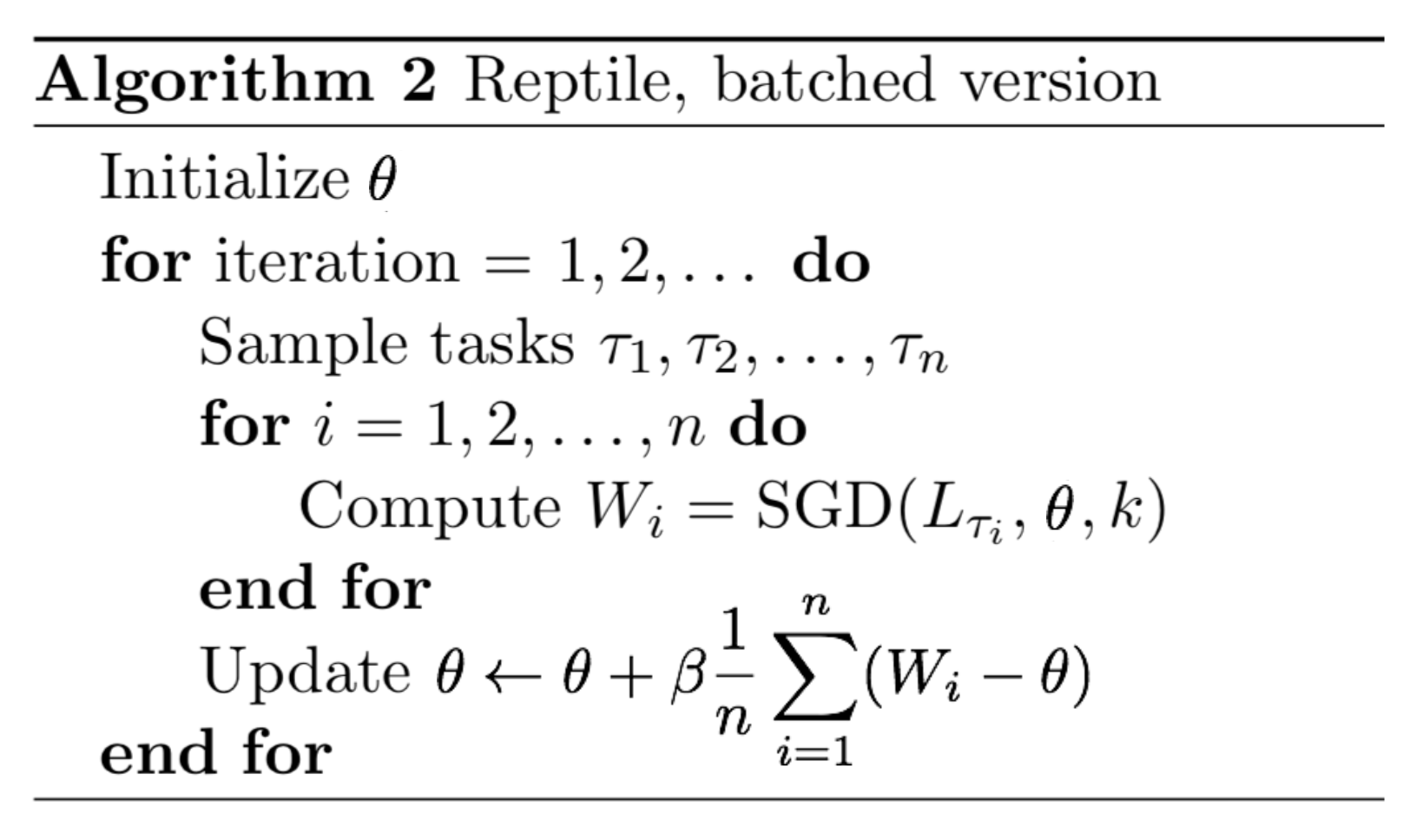Fig. 13. Batch 版本的 Reptile 算法. (图像来源：原论文)

#### The Optimization Assumption

$\theta^* = \arg\min_\theta \mathbb{E}_{\tau \sim p(\tau)} [\frac{1}{2} \text{dist}(\theta, \mathcal{W}_\tau^*)^2]$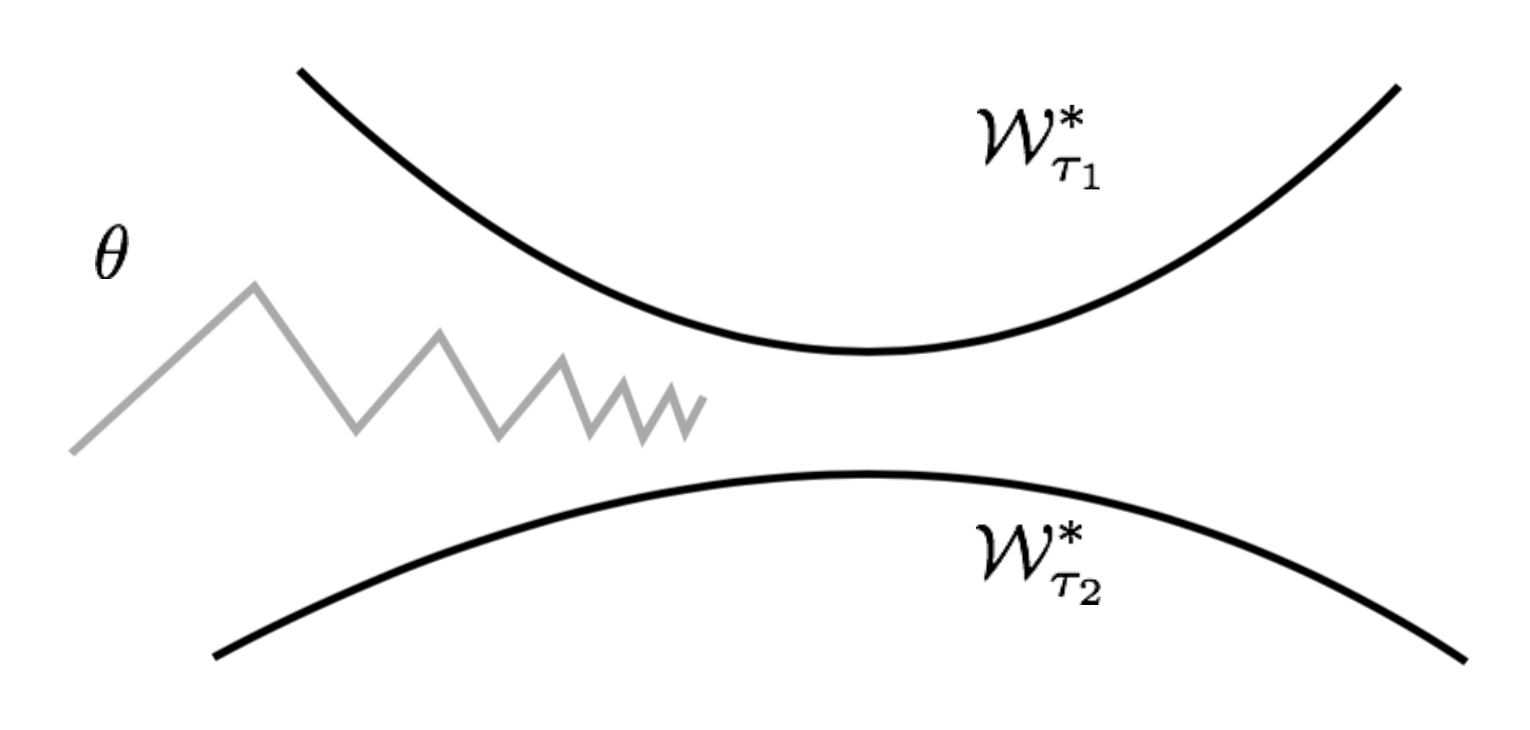Fig. 14. 为了靠近不同任务的最优流形，Reptile 算法在交替更新参数。 (图像来源：原论文)

$\text{dist}(\theta, \mathcal{W}_{\tau}^*) = \text{dist}(\theta, W_{\tau}^*(\theta)) \text{, where }W_{\tau}^*(\theta) = \arg\min_{W\in\mathcal{W}_{\tau}^*} \text{dist}(\theta, W)$

\begin{aligned} \nabla_\theta[\frac{1}{2}\text{dist}(\theta, \mathcal{W}_{\tau_i}^*)^2] &= \nabla_\theta[\frac{1}{2}\text{dist}(\theta, W_{\tau_i}^*(\theta))^2] & \\ &= \nabla_\theta[\frac{1}{2}(\theta - W_{\tau_i}^*(\theta))^2] & \\ &= \theta - W_{\tau_i}^*(\theta) & \scriptstyle{\text{; See notes.}} \end{aligned}

$\theta = \theta - \alpha \nabla_\theta[\frac{1}{2} \text{dist}(\theta, \mathcal{W}_{\tau_i}^*)^2] = \theta - \alpha(\theta - W_{\tau_i}^*(\theta)) = (1-\alpha)\theta + \alpha W_{\tau_i}^*(\theta)$

#### Reptile vs FOMAML

\begin{aligned} \theta_0 &= \theta_\text{meta}\\ \theta_1 &= \theta_0 - \alpha\nabla_\theta\mathcal{L}^{(0)}(\theta_0)= \theta_0 - \alpha g^{(0)}_0 \\ \theta_2 &= \theta_1 - \alpha\nabla_\theta\mathcal{L}^{(1)}(\theta_1) = \theta_0 - \alpha g^{(0)}_0 - \alpha g^{(1)}_1 \end{aligned}

\begin{aligned} g_\text{FOMAML} &= \nabla_{\theta_1} \mathcal{L}^{(1)}(\theta_1) = g^{(1)}_1 \\ g_\text{MAML} &= \nabla_{\theta_1} \mathcal{L}^{(1)}(\theta_1) \cdot (I - \alpha\nabla^2_{\theta} \mathcal{L}^{(0)}(\theta_0)) = g^{(1)}_1 - \alpha H^{(0)}_0 g^{(1)}_1 \end{aligned}

Reptile的梯度则定义为：

$g_\text{Reptile} = (\theta_0 - \theta_2) / \alpha = g^{(0)}_0 + g^{(1)}_1$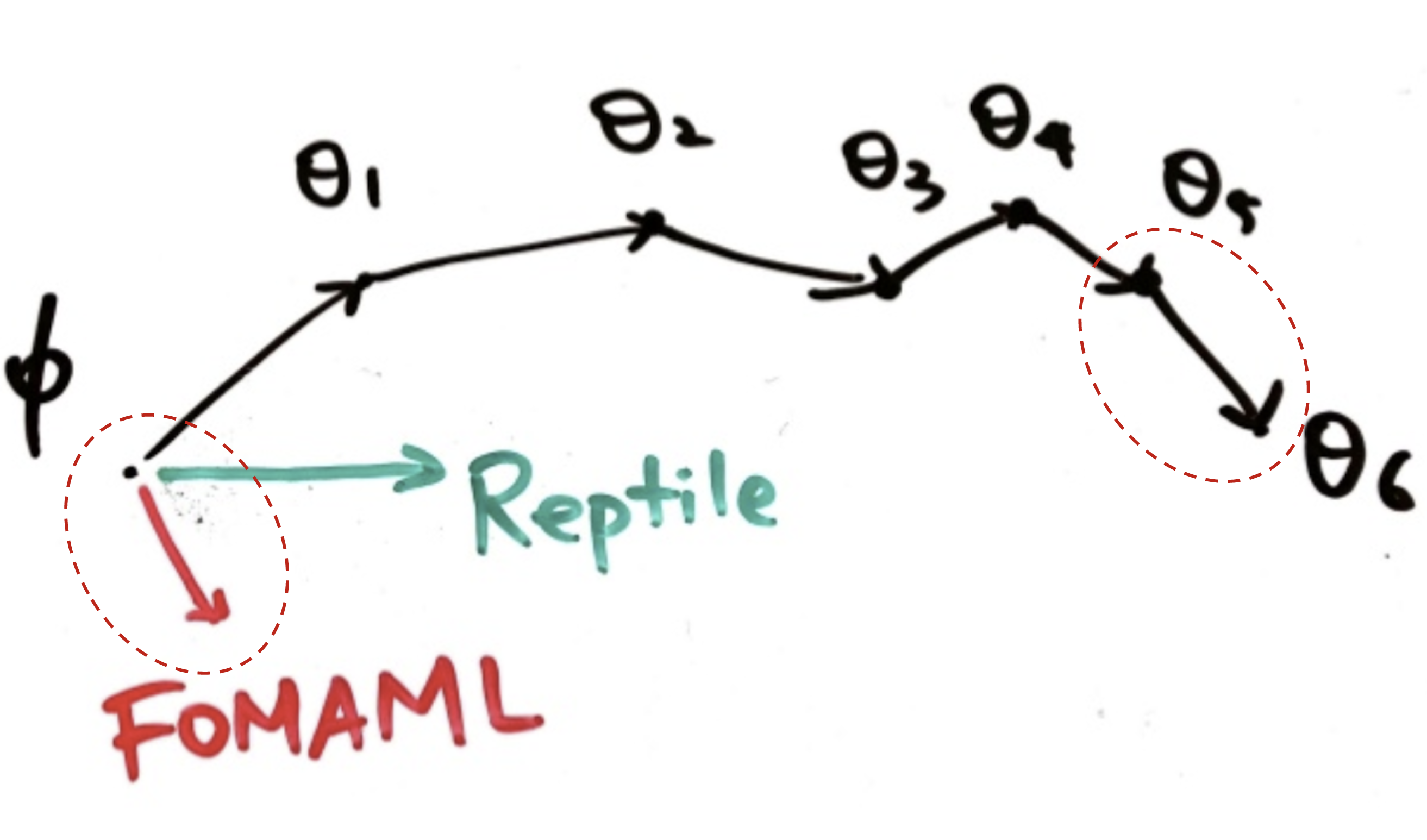Fig. 15. Reptile 和 FOMAML 在一次外循环里的元优化方式对比. (图像来源：slides on Reptile by Yoonho Lee.)

\begin{aligned} g_\text{FOMAML} &= g^{(1)}_1 \\ g_\text{MAML} &= g^{(1)}_1 - \alpha H^{(0)}_0 g^{(1)}_1 \\ g_\text{Reptile} &= g^{(0)}_0 + g^{(1)}_1 \end{aligned}

$f(x) = f(a) + \frac{f'(a)}{1!}(x-a) + \frac{f''(a)}{2!}(x-a)^2 + \dots = \sum_{i=0}^\infty \frac{f^{(i)}(a)}{i!}(x-a)^i$

\begin{aligned} g_1^{(1)} &= \nabla_{\theta}\mathcal{L}^{(1)}(\theta_1) \\ &= \nabla_{\theta}\mathcal{L}^{(1)}(\theta_0) + \nabla^2_\theta\mathcal{L}^{(1)}(\theta_0)(\theta_1 - \theta_0) + \frac{1}{2}\nabla^3_\theta\mathcal{L}^{(1)}(\theta_0)(\theta_1 - \theta_0)^2 + \dots & \\ &= g_0^{(1)} - \alpha H^{(1)}_0 g_0^{(0)} + \frac{\alpha^2}{2}\nabla^3_\theta\mathcal{L}^{(1)}(\theta_0) (g_0^{(0)})^2 + \dots & \scriptstyle{\text{; because }\theta_1-\theta_0=-\alpha g_0^{(0)}} \\ &= g_0^{(1)} - \alpha H^{(1)}_0 g_0^{(0)} + O(\alpha^2) \end{aligned}

\begin{aligned} g_\text{FOMAML} &= g^{(1)}_1 = g_0^{(1)} - \alpha H^{(1)}_0 g_0^{(0)} + O(\alpha^2)\\ g_\text{MAML} &= g^{(1)}_1 - \alpha H^{(0)}_0 g^{(1)}_1 \\ &= g_0^{(1)} - \alpha H^{(1)}_0 g_0^{(0)} + O(\alpha^2) - \alpha H^{(0)}_0 (g_0^{(1)} - \alpha H^{(1)}_0 g_0^{(0)} + O(\alpha^2))\\ &= g_0^{(1)} - \alpha H^{(1)}_0 g_0^{(0)} - \alpha H^{(0)}_0 g_0^{(1)} + \alpha^2 \alpha H^{(0)}_0 H^{(1)}_0 g_0^{(0)} + O(\alpha^2)\\ &= g_0^{(1)} - \alpha H^{(1)}_0 g_0^{(0)} - \alpha H^{(0)}_0 g_0^{(1)} + O(\alpha^2) \end{aligned}

Reptile的梯度变为：

\begin{aligned} g_\text{Reptile} &= g^{(0)}_0 + g^{(1)}_1 \\ &= g^{(0)}_0 + g_0^{(1)} - \alpha H^{(1)}_0 g_0^{(0)} + O(\alpha^2) \end{aligned}

\begin{aligned} g_\text{FOMAML} &= g_0^{(1)} - \alpha H^{(1)}_0 g_0^{(0)} + O(\alpha^2)\\ g_\text{MAML} &= g_0^{(1)} - \alpha H^{(1)}_0 g_0^{(0)} - \alpha H^{(0)}_0 g_0^{(1)} + O(\alpha^2)\\ g_\text{Reptile} &= g^{(0)}_0 + g_0^{(1)} - \alpha H^{(1)}_0 g_0^{(0)} + O(\alpha^2) \end{aligned}

• $$A = \mathbb{E}_{\tau,0,1} [g_0^{(0)}] = \mathbb{E}_{\tau,0,1} [g_0^{(1)}]$$ ; 代表着任务loss的平均梯度。沿着 $$A$$ 代表的方向更新模型，能够使模型在当前任务上的表现更好。
• $$B = \mathbb{E}_{\tau,0,1} [H^{(1)}_0 g_0^{(0)}] = \frac{1}{2}\mathbb{E}_{\tau,0,1} [H^{(1)}_0 g_0^{(0)} + H^{(0)}_0 g_0^{(1)}] = \frac{1}{2}\mathbb{E}_{\tau,0,1} [\nabla_\theta(g^{(0)}_0 g_0^{(1)})]$$ ; 代表着增加同个任务上两个小batch梯度的内积的方向。沿着 $$B$$ 代表的方向更新模型，能够使得模型在当前任务上具有更好的泛化性。

\begin{aligned} \mathbb{E}_{\tau,1,2}[g_\text{FOMAML}] &= A - \alpha B + O(\alpha^2)\\ \mathbb{E}_{\tau,1,2}[g_\text{MAML}] &= A - 2\alpha B + O(\alpha^2)\\ \mathbb{E}_{\tau,1,2}[g_\text{Reptile}] &= 2A - \alpha B + O(\alpha^2) \end{aligned}

@article{weng2018metalearning,
title   = "Meta-Learning: Learning to Learn Fast",
author  = "Weng, Lilian",
journal = "lilianweng.github.io/lil-log",
year    = "2018",
url     = "http://lilianweng.github.io/lil-log/2018/11/29/meta-learning.html"
}


## 引用

 Brenden M. Lake, Ruslan Salakhutdinov, and Joshua B. Tenenbaum. “Human-level concept learning through probabilistic program induction.” Science 350.6266 (2015): 1332-1338.

 Oriol Vinyals’ talk on “Model vs Optimization Meta Learning”

 Gregory Koch, Richard Zemel, and Ruslan Salakhutdinov. “Siamese neural networks for one-shot image recognition.” ICML Deep Learning Workshop. 2015.

 Oriol Vinyals, et al. “Matching networks for one shot learning.” NIPS. 2016.

 Flood Sung, et al. “Learning to compare: Relation network for few-shot learning.” CVPR. 2018.

 Jake Snell, Kevin Swersky, and Richard Zemel. “Prototypical Networks for Few-shot Learning.” CVPR. 2018.

 Adam Santoro, et al. “Meta-learning with memory-augmented neural networks.” ICML. 2016.

 Alex Graves, Greg Wayne, and Ivo Danihelka. “Neural turing machines.” arXiv preprint arXiv:1410.5401 (2014).

 Tsendsuren Munkhdalai and Hong Yu. “Meta Networks.” ICML. 2017.

 Sachin Ravi and Hugo Larochelle. “Optimization as a Model for Few-Shot Learning.” ICLR. 2017.

 Chelsea Finn’s BAIR blog on “Learning to Learn”.

 Chelsea Finn, Pieter Abbeel, and Sergey Levine. “Model-agnostic meta-learning for fast adaptation of deep networks.” ICML 2017.

 Alex Nichol, Joshua Achiam, John Schulman. “On First-Order Meta-Learning Algorithms.” arXiv preprint arXiv:1803.02999 (2018).

 Slides on Reptile by Yoonho Lee.